# Impact of Nonlinear Effects on Coherent and Delay Demodulation Optical Receivers

All nonlinear effects discussed in the impact of fiber nonlinearity tutorial in the context of intensity modulation/direct detection (IM/DD) systems have the potential of limiting the performance of coherent or self-coherent lightwave systems, depending on the optical power launched into the fiber. The impact of stimulated Brillouin scattering (SBS) depends on both the modulation format and the bit rate, and its effects on coherent systems have been studied extensively. The impact of stimulated Raman scattering on WDM coherent systems is less severe compared with the IM/DD systems if the information is encoded in the carrier phase because the Raman-induced power transfer depends only on the channel power. On the other hand, self- (SPM) and cross-phase modulations (XPM) play a much more important role because they convert intensity fluctuations into phase fluctuations. Because of the nonlinear origin of such fluctuations, the phase noise induced by them is known as the nonlinear phase noise. This section focuses mainly on this kind of noise.

#### 1. Nonlinear Phase Noise

Gordon and Mollenauer in 1990 were the first to study the impact of nonlinear phase noise on the performance of a long-haul system employing fiber amplifiers. By 1994, SPM-induced limitations for coherent systems were observed experimentally and studied in more detail theoretically. In a 1993 experiment that employed synchronous heterodyne detection of a BPSK signal at a bit rate of 8 Gb/s, a drop in the total transmitted distance was observed at average input power levels as low as 1 mW. With the revival of interest in phase-encoded formats after 2001, nonlinear phase noise attracted renewed attention, and by now its properties have been studied quite thoroughly.

The origin of nonlinear phase noise is easy to understand from the nonlinear optical effects tutorial where the SPM-induced nonlinear phase shift has been analyzed. In general, one must solve the nonlinear Schrödinger (NLS) equation given below numerically to see how the complex amplitude A(z, t) of the optical signal evolves inside the fiber:However, this equation can be solved analytically in the limit of negligible dispersive effects (β2 ≈ 0), and the solution for a fiber of length L is given by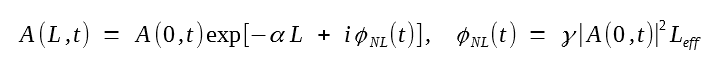where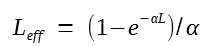is the effective fiber length defined earlier in the nonlinear optical effects tutorial. For fiber lengths longer than 50 km, we can use the approximation Leff = 1/α, where α is the fiber-loss parameter at the signal wavelength. The nonlinear parameter γ is around 2 W-1/km for telecommunication fibers in the wavelength region near 1.55 μm.

If the input field is noisy because of amplifier noise added to it at preceding fiber spans, i.e., A(0, t) = As(t) + n(t), it follows from the equation above that fluctuations in the signal phase φs are enhanced inside the fiber because of the nonlinear phase shift φNL. This enhancement is also evident in the following figure where the initial noise n(t) is shown by a circular cloud around the signal-field vector As(t). SPM inside the fiber distorts the circular cloud into an elongated ellipse because a positive amplitude fluctuation leads to a larger nonlinear phase shift than the negative one. Mathematically, with the notation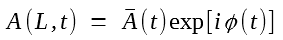the phase at the end of the fiber is given by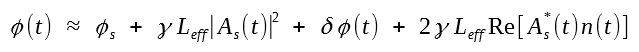where we have neglected a second-order noise term containing n2(t). The second term in this expression represents a deterministic, nonlinear shift in the signal phase that does not vary from one symbol to next and cancels out during differential detection. It represents the average value of the nonlinear phase shift. The third term represents a linear phase noise that occurs even in the absence of SPM. The last term shows how the combination of amplifier noise and SPM leads to enhanced fluctuations in the signal phase. While the initial amplifier noise is of additive nature, nonlinear phase noise is multiplicative in the equation above. It is also "colored" because of a time-dependent signal. These features indicate that the nonlinear phase noise may not remain Gaussian even if n(t) itself follows Gaussian statistics.The analysis of nonlinear phase noise is much more complicated for long-haul systems that compensate fiber losses periodically using a lumped or distributed amplification scheme. In general, one must solve the stochastic NLS equation given in the following equation (refer to compensation of fiber losses tutorial) along the entire fiber link to find the statistical properties of the optical field reaching the receiver, a challenging task even numerically:The situation becomes simpler if we neglect fiber dispersion (β2 = 0) and assume that a lumped amplifier, with the gain GA = eαL, compensates losses after every fiber span of length L. Every amplifier adds ASE noise that affects the signal and contributes to the nonlinear phase noise until the end of the entire fiber link. Since the nonlinear phase shift in each fiber section is additive, for a chain of N amplifiers, the nonlinear phase given in the equation above becomes:where nj(t) is the noise added by the jth amplifier. This expression can be used to find the probability density function (PDF) of the nonlinear phase noise after noting that the ASE noise added by one amplifier is independent of that added by other amplifiers.

As seen in the first equation, the signal phase contains two noise terms representing linear and nonlinear phase fluctuations. In practice, the PDF of the total phase φ(t) is of more interest because it controls the BER of a phase-encoded lightwave system. This case was studied in 1994 by Mecozzi, who succeeded in finding an approximate analytic expression for the BER in the case of the DPSK format. An analytic expression for the PDF of the signal phase was found by 2004 in the form of an infinite series containing hypergeometric functions.

The PDF of phase noise can be used to calculate its variance. Neglecting the impact of intensity fluctuations and making some reasonable approximations, the phase variance is given by a remarkably simple expressionwhere SASE is the ASE spectral density of noise given in the erbium-doped fiber amplifiers tutorial, LT is the total link length, E0 is the pulse energy within the symbol slot, and P0 is the corresponding peak power. The first term, representing the contribution of δφ, grows linearly with LT. The second term, having its origin in the nonlinear phase noise, grows cubically with LT and shows why SPM plays a detrimental role in phase-encoded lightwave systems. The phase variance can be minimized by optimizing P0, or the average value ⟨φNL⟩ of the nonlinear phase shift. It is easy to show by differentiating that the optimum value of ⟨φNL⟩ is √3/2.

The following figure shows three examples of the phase PDFs for optical SNR values of 10, 15, and 20 dB for the received signal. In each case, the dotted curve shows a Gaussian distribution with the same variance. Although the PDF of phase fluctuations becomes close to a Gaussian for SNR of 20 dB or more, it deviates from it for lower SNR values, especially in tails of the PDF that are important for estimating the BER. Nevertheless, the variance of phase fluctuations provides a rough measure of the impact of nonlinear phase noise on system performance and is often used as a guideline.#### 2. Effect of Fiber Dispersion

The preceding analysis of nonlinear phase noise is approximate as it neglects fiber dispersion. It is difficult to make much analytic progress because of the stochastic nature of the NLS equation: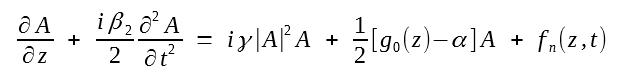However, the variance of phase noise can be calculated analytically if we employ the variational formalism or the moment method of the tutorial on role of dispersive and nonlinear effects. Such an approach also allows one to take into account variations of the loss, dispersion, and nonlinear parameters along the fiber link that occur in a dispersion-managed fiber link.

One of the conclusions of such an approach is that the variance of phase fluctuations decreases as the dispersive effects become more and more dominant. This is not surprising if we note that dispersion causes optical pulses to spread, resulting in reduced peak power, and thus a reduced nonlinear phase noise. This argument also indicates that the best system design from the standpoint of the nonlinear phase is one in which the entire dispersion is compensated at the receiver end. However, these arguments ignore the intrachannel XPM and FWM effects discussed in the pseudo-linear lightwave systems tutorial. As the dispersive effects broaden optical pulses, pulses in neighboring symbol slots begin to overlap and interact through XPM, a phenomenon that also leads to nonlinear phase noise. When the intrachannel XPM effects are taken into account properly, the variance of phase fluctuations does not decrease much with an increase in fiber dispersion. However, what matters in the case of the RZ-DBPSK format is the variance of the phase difference for the two neighboring RZX pulses. A detailed analysis shows that this variance becomes relatively small for the DBPSK format because of a partial correlation between phase noises of adjacent pulses. In this situation, the nonlinear phase noise resulting from intrachannel FWM provides the dominant contribution in the limit of large fiber dispersion.

The XPM-induced nonlinear phase noise is also important for WDM systems in which optical pulses belonging to different channels overlap periodically as they propagate inside the fiber at different speeds. This case has been analyzed using a perturbation approach. The following figure compares predicted BERs for a WDM DBPSK-format system as the number of channels increases from 1 to 49 for three dispersion-management schemes. The 12.5-Gb/s channels (25-GHz channel spacing) are transmitted over 1600 km using 20 amplifiers separated by 80 km. Each fiber span consists of standard fibers with the parameters α = 0.25 dB/km, β2 = -21.6 ps2/km, and γ = 2 W-1/km. Dispersion is compensated at amplifier locations by (a) 95%, (b) 100%, and (c) 0%; remaining dispersion compensated at the receiver. In the case of (a), BER of all channels can be reduced to near a 10-9 level by optimizing channel powers closed to 2 mW. In the case of 100% compensation (b), the interchannel XPM effects degrade the signal phase to the extent that a BER of at most 10-5 is realized even when channel powers are suitably optimized to near 1 mW. The entire dispersion is compensated once at the receiver end in (c). The BER improves in this case considerably but the optimum channel power decreased as the number of channels increases. Moreover, BER is very sensitive to this optimum value and decreases rapidly with small changes around it. In all cases, the situation is much worse for the DQPSK format. These results indicate that interchannel XPM effects limit the performance of phase-encoded WDM systems quite severely.#### 3. Compensation of Nonlinear Phase Noise

Given the serious impact of nonlinear phase noise on phase-encoded lightwave systems, the question is whether one can compensate for it with a suitable scheme. The answer turns out to be: yes, to some extent. Several compensation schemes have been proposed in recent years with varying degrees of success.

The basic reason why nonlinear phase noise can be compensated is easily understood from the equation:It shows that the nonlinear phase shift is actually a deterministic process in the sense that it is linearly proportional to the instantaneous optical power. The only reason for this phase shift to be noisy is that the power itself is fluctuating. Thus, a simple approach to nonlinear phase compensation consists of using a nonlinear device that imposes a negative phase shift on the incoming optical signal in proportion to the optical power. In essence, such a device exhibits a negative value of the nonlinear parameter γ. In a 2002 proposal, this device was in the form of a periodically poled LiNbO3 waveguide that provided a negative nonlinear phase shift through a cascaded second-order nonlinear process. Such a scheme is referred to as post-nonlinearity compensation because it is implemented at the end of the fiber link.

In another implementation of this idea, optical power is first detected at the receiver end, and the resulting current is used to drive a LiNbO3 phase modulator that imposes a negative phase shift proportional to the optical power on the received optical signal. This scheme was implemented in a 2002 experiment using two phase modulators that processed orthogonally polarized components of the signal to impose a polarization-independent phase shift on the signal. The compensation of SPM-induced phase shift was observed through a reduction in spectral broadening.

Extensive numerical simulations performed for single-channel and WDM systems indicate that a post-nonlinearity compensation scheme reduces the nonlinear phase noise but does not eliminate it. The reason can be understood from the following equation: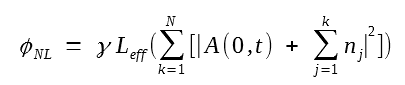It shows that the nonlinear phase shift builds up along the fiber link, with the noise added by multiple amplifiers. Since intensity noise itself evolves along the fiber link, the use of optical power at the end of the link cannot cancel the nonlinear phase noise completely. As an example, the figure below shows, using phasor diagrams, the extent of phase-noise reduction after 6000-km transmission of DBPSK signals for WDM channels operating at 10 Gb/s. The dispersion-managed link consisted of 100-km fiber spans (D = 6 ps/km/nm) with partial dispersion compensation at amplifiers, a pre-dispersion of 2300 ps/nm, and a post-dispersion of 150 ps/nm. Fiber losses of 23 dB during each span were assumed to be compensated through backward Raman amplification. This figure shows that the effectiveness of the compensation scheme is reduced for closely spaced channels. The reason is related to the interchannel XPM process that generates additional nonlinear phase noise. This XPM-induced noise can be reduced by adopting the polarization interleaving technique that ensures that neighboring channels are orthogonally polarized.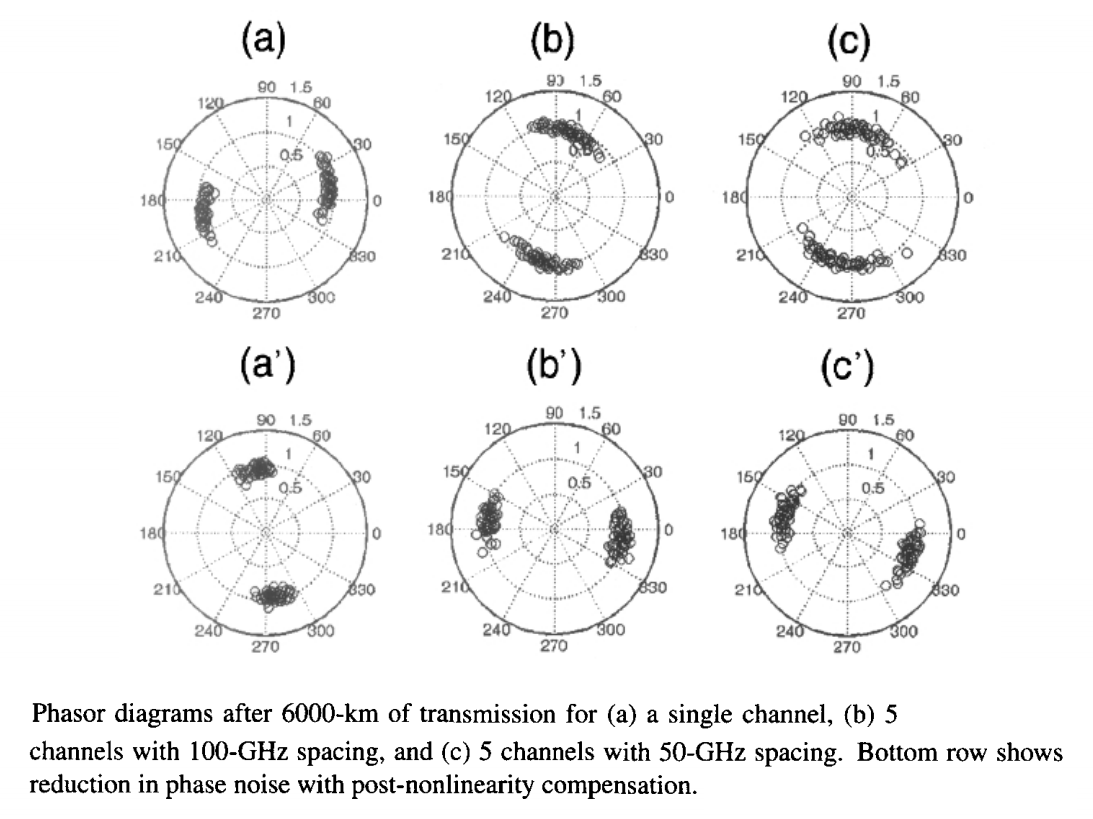The use of a phase modulator, driven by a current proportional to received optical power also reduces the nonlinear phase noise. However, one needs to optimize the fraction of received power that is sent to the detector. Also, one must ask whether the phase modulator should be used at the end of the fiber link or placed within the link at an optimum distance. These issues have been analyzed and it is found that the phase variance can be reduced by a factor of 9 by placing the phase modulator at a distance of 2LT/3, where LT is the total link length. Even larger reduction factors are possible by placing two or more phase modulators at optimum locations within the fiber link. On the experimental side, a single phase modulator, placed at the end of a 1-km-long highly nonlinear fiber, reduced the phase noise of a single 10-Gb/s DBPSK channel considerably, resulting in a higher electrical SNR and an improved eye diagram.

Another scheme for phase-noise compensation makes use of optical phase conjugation (OPC). As already discussed in the optical phase conjugation tutorial, OPC can compensate the GVD and SPM effects simultaneously. OPC can also compensate for the timing jitter induced by amplifier noise. Thus, it is not surprising that OPC is suitable for compensation of SPM-induced nonlinear phase noise. The only question is at what location along the fiber link should be phase conjugator be placed and to what extent is the phase noise compensated. As we saw in the optical phase conjugation tutorial, dispersion compensation requires mid-span placement of the OPC device. However, such a choice is not optimum for phase-noise compensation. Nonlinear phase noise builds up along the fiber link such that the second half of the fiber link contributes to it much more than the first half. Clearly, it is better if OPC is carried out in the second half of the fiber link.

The variance σ2φ of nonlinear phase noise and its reduction with OPC can be calculated using a variational, moment, or perturbation method. These methods can also be used to find the optimum location of the phase conjugator. The following figure shows how much the OPC helps to reduce the phase variance for two different locations of the phase conjugator. When it is placed exactly at the mid-point, the phase variance is reduced by a factor of 4 (or 6 dB). However, it can be reduced by 9.5 dB, or almost by a factor of 10, when the phase conjugator is placed at a distance of 0.66LT. Even larger reductions are possible by using two phase conjugators. A 12-dB reduction in phase variance occurs when they are placed at the distance of LT/4 and 3LT/4. This value can be increased to 14 dB by placing the phase conjugators at 40% and 80% of the total link length. One should keep in mind that the level of dispersion compensation provided by a phase conjugator also depends on its location. For example, in the case of two phase conjugators, 100% dispersion compensation occurs in the first configuration but it is reduced to an 80% level in the second configuration.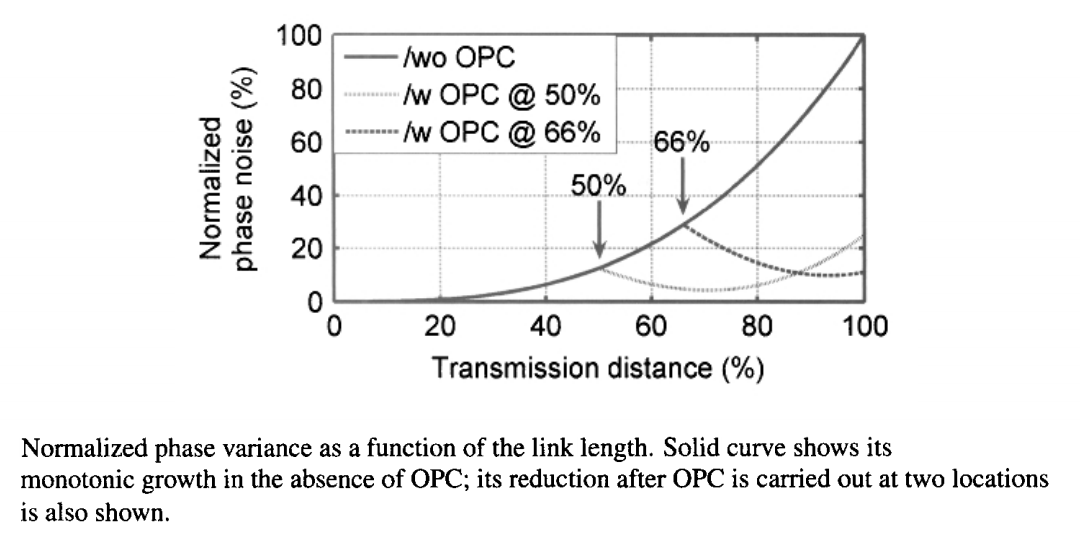Experiments have been performed to observe the OPC-induced reduction in the nonlinear phase noise. In one experiment, a single 10.7-Gb/s DBPSK channel was transmitted over 800 km, with OPC taking place at various locations of the fiber link. The performance was characterized using a Q factor defined in terms of the observed BER as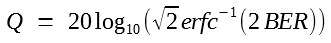This Q factor improved by 4 dB when OPC was implemented at the midpoint of the link. In this experiment, the optimum location was the midpoint as improvement in the Q factor was reduced on either side of this location.

In another WDM experiment, 44 DQPSK channels, with 50-GHz spacing and 10.7-GHz symbol rate, were transmitted over 10,200 km using a recirculating loop configuration. Losses inside fibers were compensated using EDFAs in combination with backward Raman amplification. Midpoint-OPC was carried out using a periodically poled LiNbO3 waveguide. Figure (a) below shows the Q factor as a function of distance, with and without OPC, for a typical channel. Without OPC, the Q factor began to degrade rapidly after 6000 km, and the signal could not be transmitted error-free after 7800 km. Such rapid degradation does not occur when OPC is employed, and the WDM system operates error-free over 10,200 km. Figure (b) below shows that the Q factors of all channels at a distance of 10,200 km remain above the FEC limit of 9.1 dB. These results clearly illustrate the potential of OPC-based compensation of nonlinear phase noise in real WDM systems.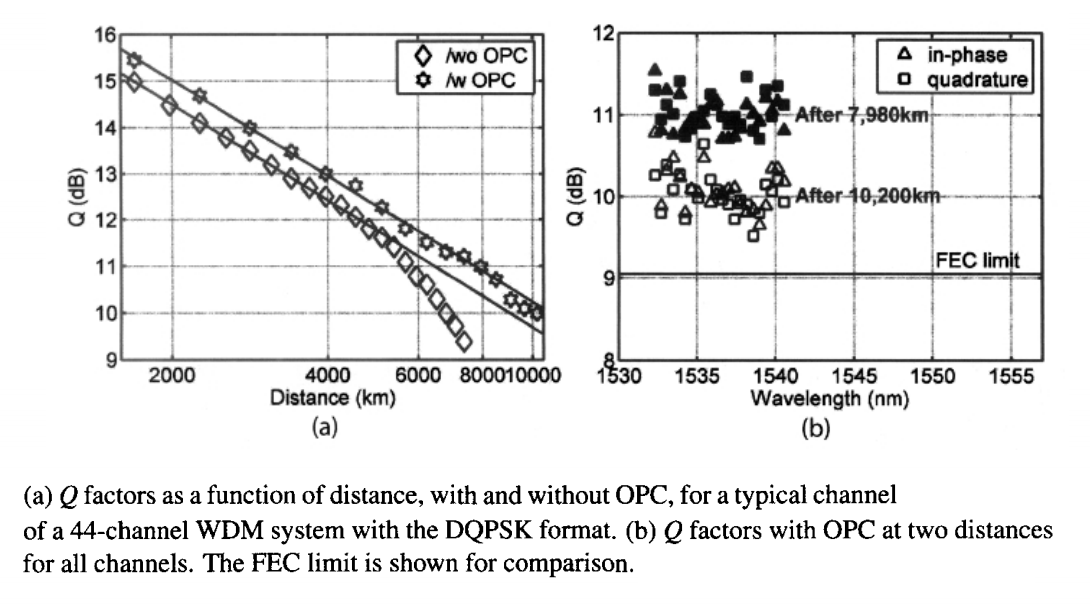Several other techniques can provide partial compensation of nonlinear phase noise. An electronic compensation scheme can be used inside the optical receiver simply by subtracting from the received phase a correction proportional to the incident power. With proper optimization, phase variance can be reduced by up to a factor of 4, resulting in a doubling of the transmission distance. Nonlinear phase noise within the fiber link can be controlled to some extent by optimizing the number and locations of in-line amplifiers. The use of Wiener filtering has also been suggested for this purpose.